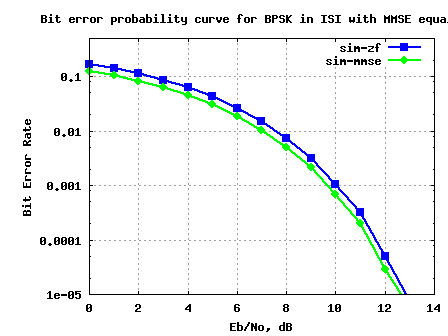- DSP log - http://www.dsplog.com -

BER for BPSK in ISI channel with MMSE equalization

Posted By Krishna Sankar On January 24, 2010 @ 11:34 am In Channel | 65 Comments

In the past, we had discussed BER for BPSK in flat fading Rayleigh channel  and BER for BPSK in a frequency selective channel using Zero Forcing Equalization . In this post, lets discuss a frequency selective channel with the use of Minimum Mean Square Error (MMSE) equalization to compensate for the inter symbol interference (ISI). For simplifying the discussion, we will assume that there is no pulse shaping at the transmitter. The ISI channel is assumed to be a fixed 3 tap channel.

Transmit symbol

Let the transmit symbols be modeled as

, where

is the symbol period,

is the symbol to transmit,

is the transmit filter,

is the symbol index and

is the output waveform.

For simplicity, lets assume that the transmit pulse shaping filter is not present, i.e .

So the transmit symbols can be modeled by the discrete time equivalentFigure: Transmit symbols

Channel Model

Lets us assume the channel to be a 3 tap multipath channel with spacing i.e.

Figure: Channel model (3 tap multipath)

In addition to the multipath channel, the received signal gets corrupted by noise , typically referred to as Additive White Gaussian Noise (AWGN). The values of the noise follows the Gaussian probability distribution function, with

mean and

variance .

, where

is the convolution operator.

MMSE Equalization

In Minimum Mean Square Error solution, for each sample time we would want to find a set of coefficients which minimizes the error between the desired signal and the equalized signal , i.e.

,

where,

is the error at sample time ,

is column vector of dimension storing the equalization coefficients,

is column vector of dimension storing the received samples,

is the number of taps in the equalizer,

is the cross correlation between received sequence and input sequence ,

is the cross correlation between received sequence and input sequence and

is the auto-correlation of the received sequence.

For solving the Minimum Mean Square Error (MMSE) criterion, we need to find a set of coefficients which minimizes .

Differentiation with respect to and equating to 0,

.

Simplifying,

,

Note :

a) is the variance of the input signal

b) (as there is no correlation between input signal and noise)

Simulation Model

The attached Matlab/Octave simulation script performs the following:

(a) Generation of random binary sequence

(b) BPSK modulation i.e bit 0 represented as -1 and bit 1 represented as +1

(c) Convolving the symbols with a 3-tap fixed fading channel.

(e) Computing the MMSE and ZF equalization filter at the receiver (with 7 taps in length)

(f) Demodulation and conversion to bits

(g) Counting the number of bit errors

(h) Repeating for multiple values of Eb/No

The simulation results are as shown in the plot below.Figure: BER plot for BPSK in a 3 tap ISI channel with MMSE equalizer

Observations

1. Can see around 0.5dB gain with using MMSE equalizer

Reference

URL to article: http://www.dsplog.com/2010/01/24/ber-bpsk-isi-channel-mmse-equalization/

URLs in this post:

 BER for BPSK in flat fading Rayleigh channel: http://www.dsplog.com/2008/08/10/ber-bpsk-rayleigh-channel/

 BER for BPSK in a frequency selective channel using Zero Forcing Equalization: http://www.dsplog.com/2009/11/29/ber-bpsk-isi-channel-zero-forcing-equalization/